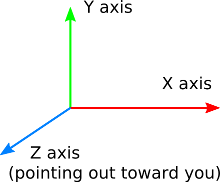# [Does my object rotate around the fixed coordinate system I defined when I use this method ?]

Hello guys,
I hope you’re doing well.
So I’m developping an application that allows a user to see the orientation of a satellite with the respect to the orbital frame.

So in my paintGL() function, I started with drawing the fixed coordinate system.
I know that the coordinate system represented in the picture below is the OpenGL standard coordinate system:But I wanted to change the orientation of its axes orientation using transformations, more precisely rotations as follows:

``````     QMatrix4x4 localMatrix;

glMatrixMode(GL_PROJECTION);

glMatrixMode(GL_MODELVIEW);
gluLookAt(2.0,2.0,0.0, 0.0,0.0,-5.0,0.0,1.0,0.0);

glTranslatef(0.0,0.0,-5.0);

glRotatef(180.0,0.0,1.0,0.0); // changing the axes orientation
glRotatef(-90.0,1.0,0.0,0.0); // changing the axes orientation

glScalef(0.4,0.4,0.4);

DrawOrbitalFrame();
``````

Then I drew my object using shaders:

`````` program.bind();

texture->bind();
localMatrix.setToIdentity();
localMatrix.lookAt(QVector3D(2.0, 2.0, 0.0),
QVector3D(0.0,0.0,-5.0),QVector3D(0.0,1.0,0.0));

localMatrix.translate(0.0, 0.0, -5.0);

localMatrix.scale(0.4,0.4,0.4);

quaternion = QQuaternion(quat_w, quat_x, quat_y, quat_z);

localMatrix.rotate(180.0,0.0,1.0,0.0);
localMatrix.rotate(-90.0,1.0,0.0,0.0);

localMatrix.rotate(quaternion);

quaternion=csvquaternion;
localMatrix.rotate(quaternion);

program.setUniformValue("mvp_matrix", projection * localMatrix);
update();
// Use texture unit 0 which contains cube.png
program.setUniformValue("texture", 0);

// Draw cube geometry
geometries->drawCubeGeometry(&program);

texture->release();
//for (int j=0; j<6; ++j) {textures[j]->release();}

program.release();

``````

then right after I drew a coordinate system which is attached to the satellite ( the satellite body frame).
My goal is to rotate the satellite using quaternion with respect to the orbital frame.
When I set the quaternion values as follows: w=0.7, x=0.0, y=0.07, z=0.0, the object is supposed to rotate aroundthe y-axis of the fixed coordinate system( the orbital frame) with 90°.
It does rotate but around the y-axis of the OpenGL standard coordinate system and not the one on which I applied the transformations to change its axes orientation.

So as a solution to this problem, before drawing the object and rotating it using quaternion, I applied the same transformations as I did before drawing the fixed coordinate system ( orbital frame), as shown in the code lines above.

Now the object seems to rotate around the y-axis of the orbital frame however I’m not sure this is really correct.
Can someone please tell me if I’m on the right path ?

This topic was automatically closed 183 days after the last reply. New replies are no longer allowed.